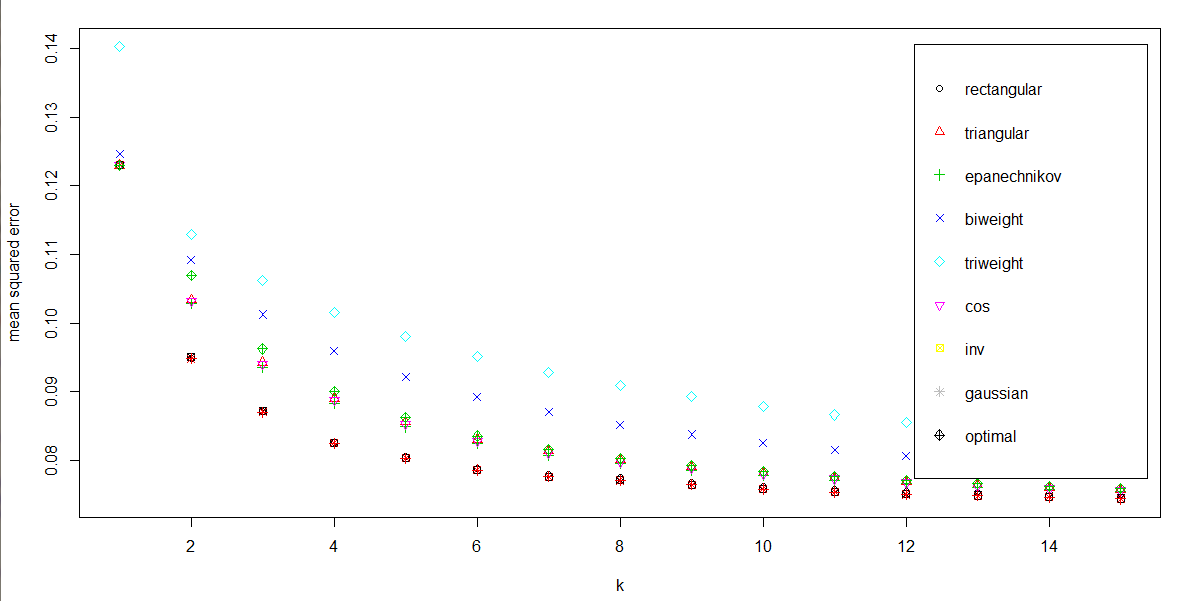## Tuesday, 6 May 2014

### R Code - Bank Subscription Marketing - Classification {K Nearest Neighbour}

R Code  for K Nearest Neighbour (KNN)
Data Set:- Bank Marketing https://www.blogger.com/blogger.g?blogID=4359514443959080595#editor/target=post;postID=5426750358330739357
Source of Data Set:-  UCI Repository (http://archive.ics.uci.edu/ml/datasets/Bank+Marketing)
The Code includes the following:-
1. Data Exploration - Missing Values, Outliers
2. Data Visualisation
3.  Correlation Matrix
4. Data Partitioning
5. Package used "KKNN"
6. Plots
7. Confusion Matrix

## The data has been imported using Import Dataset option in R Environment
## The data set can be obtained from http://archive.ics.uci.edu/ml/datasets/Bank+Marketing
## DATASET UNDERSTANDING
head(bank_full) ## Displays first 6 rows for each variable
str(bank_full) ## Describes each variables
summary(bank_full) ## Provides basic statistical information of each variable

## DATA EXPLORATION - Check for Missing Data
## Option 1
is.na(bank_full) ## Displays True for a missing value
## Since it is a large dataset, graphical display of missing values will prove to be easier
##Option 2
require(Amelia)
missmap(bank_full,main="Missing Data - Bank Subscription", col=c("red","grey"),legend=FALSE)

## No red colour stripes are visible. hence no missing values.
##Option 3
summary(bank_full) ## displays missing values if any under every variable

## DATA VISUALISATION
## Use Box plots (Only for continuous variables)- To Check Ouliers
boxplot(bank_full\$age~bank_full\$subscribed, main=" AGE",ylab="age of customers",xlab="Subscribed")
boxplot(bank_full\$balance~bank_full\$subscribed, main=" BALANCE",ylab="Balance of customers",xlab="Subscribed")
boxplot(bank_full\$lastday~bank_full\$subscribed, main=" LAST DAY",ylab="Last day of contact",xlab="Subscribed")
boxplot(bank_full\$lastduration~bank_full\$subscribed, main="LAST DURATION",ylab="Last duration of contact",xlab="Subscribed")
boxplot(bank_full\$numcontacts~bank_full\$subscribed, main="NUM CONTACTS",ylab="number of contacts",xlab="Subscribed")
boxplot(bank_full\$pdays~bank_full\$subscribed, main=" Previous DAYS",ylab="Previous days of contact",xlab="Subscribed")
boxplot(bank_full\$pcontacts~bank_full\$subscribed, main=" Previous Contacts",ylab="Previous Contacts with customers",xlab="Subscribed")

## Though some outliers are observed in Previous contacts, NumContacts and LastDuration, they have not bee removed keeping their significance into consideration
## Use Histograms (For both continuous and categorical variables)
## These histograms provide details abpout Skewness, Normal Distribution etc
## Function to create histograms for continuous variables with normal curve
bank_Conthist<-function(VarName,NumBreaks,xlab,main,lengthxfit) ## xlab and main should be mentioned under quotes as they are characters
{
hist(VarName,breaks=NumBreaks,col="yellow",xlab=xlab,main=main)
xfit<-seq(min(VarName),max(VarName),length=lengthxfit)
yfit<-dnorm(xfit,mean=mean(VarName),sd=sd(VarName))
yfit<-yfit*diff(h\$mids[1:2])*length(VarName)
lines(xfit,yfit,col="red",lwd=3)
}
bank_Conthist(bank_full\$age,10,"age of customers","AGE",30)
bank_Conthist(bank_full\$balance,50,"Balance of customers","Balance",100)

## Balance is more skewed towards to Negative or Zero
bank_Conthist(bank_full\$lastday,5,"Last Day of contact","LAst Day",10)
bank_Conthist(bank_full\$lastduration,100,"LastDuration of COntact","Last Duration",10)

## Last Duration is more skewed towards 0 to 100 secs.
bank_Conthist(bank_full\$numcontacts,30,"Number of Contacts","NUmContacts",20)
## NUmContacts are more skewed towards 1
bank_Conthist(bank_full\$pdays,30,"Previous Days of contacts","PDays",20)
## Many were not contacted previously
bank_Conthist(bank_full\$pcontacts,20,"Previous Contacts","PContacts",10)
## Since many were not contacted previously, therefore Pcontacts is 0

## Barplots for Categorical Variables
barplot(table(bank_full\$job),col="red",main="JOB")
barplot(table(bank_full\$marital),col="green",main="Marital")
barplot(table(bank_full\$education),col="red",main="Education")
barplot(table(bank_full\$creditdefault),col="red",main="Credit Default")

## Since Credit Default is highly skewed towards NO, this shall be removed from further analysis
bank_full<-NULL
str(bank_full)
barplot(table(bank_full\$housingloan),col="red",main="Housing Loan")
barplot(table(bank_full\$personalloan),col="blue",main="Personal Loan")
barplot(table(bank_full\$lastcommtype),col="red",main="Last communication type")
barplot(table(bank_full\$lastmonth),col="violet",main="Last Month")
barplot(table(bank_full\$poutcome),col="magenta",main="Previous Outcome")

## Correlation Matrix among input (or independent) continuous variables
bank_full.cont<-data.frame(bank_full\$age,bank_full\$balance,bank_full\$lastday,bank_full\$lastduration,bank_full\$numcontacts,bank_full\$pdays,bank_full\$pcontacts)
str(bank_full.cont)
cor(bank_full.cont)

## It can be observed that No two variables are highly correlated

## CLASSIFICATION USING K Nearest Neighbour (KNN) Model
install.packages("kknn")
library(kknn)

## Prior running the KNN model, the dataset has top be transformed to Numeric or integral as shown below
## One cannot use directly as.numeric() to convert factors to numeric as it has limitations.
## First, Lets convert factors having character levels to numeric levels
str(bank_full)
bank_full_transform<-bank_full
bank_full_transform\$marital=factor(bank_full_transform\$marital,levels=c("single","married","divorced"),labels=c(1,2,3))
bank_full_transform\$education=factor(bank_full_transform\$education,levels=c("primary","secondary","tertiary","unknown"),labels=c(1,2,3,4))
bank_full_transform\$housingloan=factor(bank_full_transform\$housingloan,levels=c("no","yes"),labels=c(1,2))
bank_full_transform\$personalloan=factor(bank_full_transform\$personalloan,levels=c("no","yes"),labels=c(1,2))
bank_full_transform\$subscribed=factor(bank_full_transform\$subscribed,levels=c("no","yes"),labels=c(1,2))
bank_full_transform\$lastcommtype=factor(bank_full_transform\$lastcommtype,levels=c("cellular","telephone","unknown"),labels=c(1,2,3))
bank_full_transform\$poutcome=factor(bank_full_transform\$poutcome,levels=c("failure","other","success","unknown"),labels=c(1,2,3,4))
bank_full_transform\$lastmonth=factor(bank_full_transform\$lastmonth,levels=c("apr","aug","dec","feb","jan","jul","jun","mar","may","nov","oct","sep"),labels=c(1,2,3,4,5,6,7,8,9,10,11,12))
str(bank_full_transform)

## Now convert these numerical factors into numeric
bank_full_transform\$subscribed<-as.numeric(as.character(bank_full_transform\$subscribed))
bank_full_transform\$job<-as.numeric(as.character(bank_full_transform\$job))
bank_full_transform\$marital<-as.numeric(as.character(bank_full_transform\$marital))
bank_full_transform\$education<-as.numeric(as.character(bank_full_transform\$education))
bank_full_transform\$personalloan<-as.numeric(as.character(bank_full_transform\$personalloan))
bank_full_transform\$housingloan<-as.numeric(as.character(bank_full_transform\$housingloan))
bank_full_transform\$lastcommtype<-as.numeric(as.character(bank_full_transform\$lastcommtype))
bank_full_transform\$lastmonth<-as.numeric(as.character(bank_full_transform\$lastmonth))
bank_full_transform\$poutcome<-as.numeric(as.character(bank_full_transform\$poutcome))
bank_full_transform\$age<-as.numeric(as.character(bank_full_transform\$age))
bank_full_transform\$balance<-as.numeric(as.character(bank_full_transform\$balance))
bank_full_transform\$lastday<-as.numeric(as.character(bank_full_transform\$lastday))
bank_full_transform\$lastduration<-as.numeric(as.character(bank_full_transform\$lastduration))
bank_full_transform\$numcontacts<-as.numeric(as.character(bank_full_transform\$numcontacts))
bank_full_transform\$pdays<-as.numeric(as.character(bank_full_transform\$pdays))
bank_full_transform\$pcontacts<-as.numeric(as.character(bank_full_transform\$pcontacts))
str(bank_full_transform)

## Now all the variables are wither intergers or numeric
## Partitioning Data into Train and Test datasets in 70:30
library(caret)
set.seed(1234567)
train2<-createDataPartition(bank_full_transform\$subscribed,p=0.7,list=FALSE)
train<-bank_full_transform[train2,]
test<-bank_full_transform[-train2,]

write.table(bank_full_transform,"bank1.csv",sep=",")
str(train)
str(test)

## Remember to check for missing values
which(is.na(train))
which(is.na(test))

## Spectral Clustering based on K Nearest Neighbour Graph for train Dataset
x<-specClust(train[,1:15],centers=NULL,nn=10,method="symmetric",gmax=NULL)
##Other methods like random-walk or NULL can also be used
summary(x)
x ## To know the number of clusters
plot(x)
table[train[,15],x\$cluster]

## Train the Dataset (Train) using KKNN method via leave-one-out crossvalidation
train.kknn<-train.kknn(subscribed~.,train,kmax=15,distance=1.5,kernel=c("rectangular","triangular","epanechnikov","biweight","triweight","cos","inv","gaussian","optimal"),ykernal=NULL,scale=TRUE)
## For better optimisation of your model, one can choose suitable kernal, vary the distance (which is a parameter of miniskowi) and kmax
plot(train.kknn)

## Cross validation procedure to test prediction accuracy
## Output is mean and SD of miclssification error. Hence, lower the values are much better for the model
simulation(subscribed~.,train,runs=10,kernel="triangular",k=10,train=TRUE)
## Any kernel can be used for beter modeling purposes

## For Test Data set - Accuracy prediction
train.kknn=kknn(subscribed~.,train=train,test=test,k=10,distance=1,kernel="gaussian",ykernel=NULL,scale=TRUE,kknn.dist(learn=train,valid=test,k=10,distance=1))
## misclassification table
fit=fitted(train.kknn)
table(predict(train.kknn,train),train\$subscribed)
pairs(train[,1:15],pch=train\$subscribed,col=c("green","red")[(train\$subscribed!=fit)+1])

PLOT of the Trained Model using different kernels.
Kernel having least mean squared error is selected for training the data set